# Intermediate Geometry : How to find if of acute / obtuse isosceles triangles are congruent

## Example Questions

### Example Question #48 : Acute / Obtuse Isosceles Triangles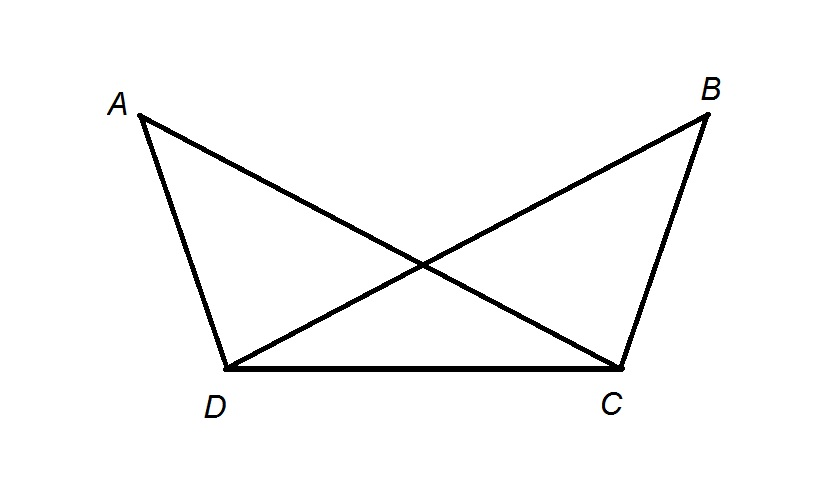Refer to the above diagram.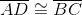and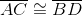. By what statement does it follow that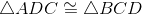The Converse of the Isosceles Triangle Theorem

The Hinge Theorem

The Side-Side-Side Postulate

The Side-Angle-Side Postulate

The Isosceles Triangle Theorem

The Side-Side-Side Postulate

Explanation:

In addition to the fact thatand, we also have that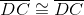, since, by the Reflexive Property of Congruence, any segment is congruent to itself. We can restate this in a more usable form as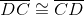; since we have three side congruences between triangles, it follows from the Side-Side-Side Postulate that.

### Example Question #49 : Acute / Obtuse Isosceles Triangles

Given: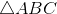and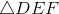such that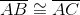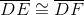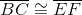Which statement(s) must be true?

(a)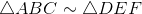(b)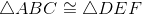(a) but not (b)

(b) but not (a)

(a) and (b)

Neither (a) nor (b)

Neither (a) nor (b)

Explanation:

Neither similarity nor congruence of the two triangles follows from the statements given, as can be seen from the figure below: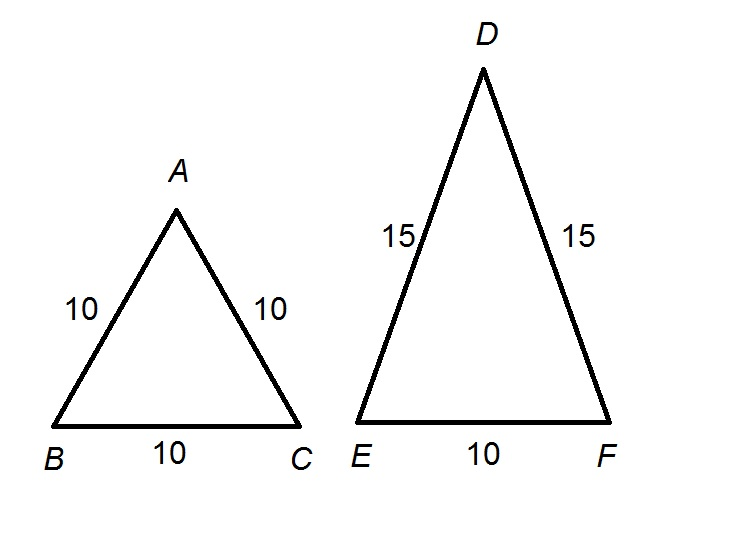, and. However, the triangles are not similar, as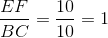and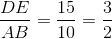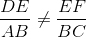, so at least one pair of corresponding sides is not in proportion. Therefore,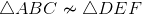The triangles are not similar, and thus cannot be congruent either, so neither statement holds.